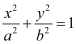Which of the following statements are true and which are false? In each case give a valid reason for saying so.

(i) p: Each radius of a circle is a chord of the circle.

(ii) q: The centre of a circle bisects each chord of the circle.

(iii) r: Circle is a particular case of an ellipse.

(iv) s: If x and y are integers such that x > y, then –x < –y.

(v) t:$$\sqrt{11}$$ is a rational number.

Asked by Pragya Singh | 1 year ago |  91

##### Solution :-

Explanation:-

According to the definition of chord, it should intersect the circle at two distinct points.

Explanation:-

If the chord is not the diameter of the circle, then the centre will not bisect that chord.

Explanation:-

The equation of an ellipse is,If we put a = b = 1, then we get

x2 + y2 = 1, which is an equation of a circle

Hence, circle is a particular case of an ellipse.

Therefore, statement r is true

Explanation:-

x > y

By a rule of inequality

-x < – y

Hence, the given statement s is true

Explanation:-

11 is a prime number and we know that the square root of any prime number is an irrational number.
Therefore, $$\sqrt{11}$$ is an irrational number. Thus, the given statement t is false.

Answered by Abhisek | 1 year ago

### Related Questions

#### Determine whether the argument used to check the validity of the following statement is correct:

Determine whether the argument used to check the validity of the following statement is correct: p: “If x2 is irrational, then x is rational.” The statement is true because the number x2 = π2 is irrational, therefore x = π is irrational.

#### Which of the following statements are true and which are false? In each case give a valid reason for saying so

Which of the following statements are true and which are false? In each case give a valid reason for saying so

(i) p: Each radius of a circle is a chord of the circle.

(ii) q: The centre of a circle bisect each chord of the circle.

(iii) r: Circle is a particular case of an ellipse.

(iv) s: If x and y are integers such that x > y, then – x < – y.

(v) t: $$\sqrt{11}$$ is a rational number.

#### By giving a counter example, show that the following statement is not true.

By giving a counter example, show that the following statement is not true. p: “If all the angles of a triangle are equal, then the triangle is an obtuse angled triangle.”